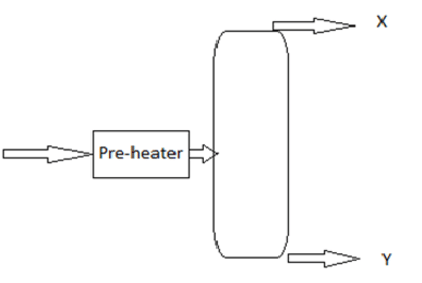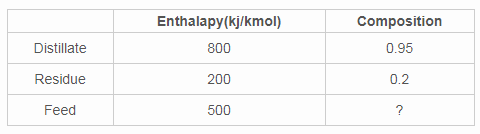# Test: Flash Vaporization

## 10 Questions MCQ Test Mass Transfer | Test: Flash Vaporization

Description
Attempt Test: Flash Vaporization | 10 questions in 20 minutes | Mock test for Chemical Engineering preparation | Free important questions MCQ to study Mass Transfer for Chemical Engineering Exam | Download free PDF with solutions
QUESTION: 1

### Flash vaporization is a

Solution:

Explanation: Since the liquids are partially vaporized and allowed to be in equilibrium with vapour.

QUESTION: 2

### The feed is directly passed to the flash vaporization column

Solution:

Explanation: The feed must be pre-heated.

QUESTION: 3

### Find the X and Y from the flash column given belowSolution:

Explanation: Since the more volatile component has the low vapour pressure it will becomes vapour and comes out in the top.

QUESTION: 4

Binary mixture of 100 kmol/hr enters into a flash drum has equimolar composition. The distillate obtained is 60 kmol/hr and the enthalpy of distillate, residue and feed are 500 KJ/kmol, 200 KJ/kmol and 400 KJ/kmol. Find the bottom flow rate in kmol/hr.

Solution:

Explanation: -W/D = HD-HF/HW-HF
Where, HD, HW,HF are enthalpy of distillate, residue and feed.
-W/60 = -0.5
W= 30

QUESTION: 5

Find the ratio of distillate to residual flow rates, if a binary mixture containing 65% of more volatile component while the distillate and the residue compositions are 90% and 30%.

Solution:

Explanation: -W/D=(xd – xf)/(xw-xf)
Finally we ger –W/D= -0.416
D/W= 2.4

QUESTION: 6

What happens to the liquid after preheating when enter to the cyclonic flash drum?

Solution:

Explanation: Since the column is like cyclone it will split the liquid with centrifugal force for the further separation.

QUESTION: 7

Express the flash vaporization

Solution:

Explanation: Only single drum is used.

QUESTION: 8

Find the heat given( in KJ) to the feed for the flash vaporization process if the ratio of residue to the distillate is 0.65 and the enthalpy of feed, distillate and the residue are 500, 800 and 200 KJ/kmol respectively. The feed rate is 100 kmol/hr.

Solution:

Explanation: -W/D = (HD-(Hf+Q/f))/ (Hw- (Hf+Q/F))
On apply the values, we get 6363 KJ

QUESTION: 9

Find the distillate and the residue rates in mole/hr, if the feed rate is 100 mol/hr; The compositions of feed, distillate and residue in terms of mole fraction are 0.65, 0.9 and 0.2.

Solution:

Explanation: F*xf= D*xd+ W*xw
On applying the values, we get distillate rate= 64 mol/hr and residue rate is 36 mol/hr.

QUESTION: 10

Find the feed composition of more volatile component by analysing the table below.Hint: can neglect pre-heater.

Solution:

Explanation: (xd- xf)/(xw- xf) =Hd- Hf/(Hw-Hf)
On applying values, we get xf= 0.575Use Code STAYHOME200 and get INR 200 additional OFF Use Coupon Code